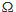# In a series LCR circuit R = 200  and the voltage and the frequency of the main supply is 220 V and 50 Hz respectively. On taking out the cap...

In a series LCR circuit R = 200and the voltage and the frequency of the main supply is 220 V and 50 Hz respectively. On taking out the capacitance from the circuit the current lags behind the voltage by 30°. On taking out the inductor from the circuit the current leads the voltage by 30°. The power dissipated in the LCR circuit is

Anonymous User Physics Electromagnetic Induction & Alternating Current 11 May, 2020 65 views